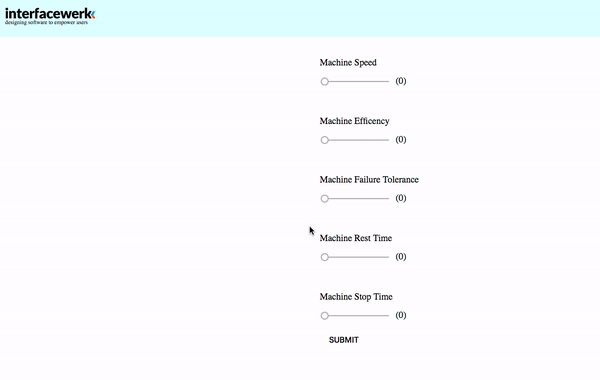# HMI form prediction in angular with artificial intelligence

In HMIs, users fill in forms to control machine behaviour. In HMI context often similar values have to be entered and setting values multiple times by hand can become a burden. We present a solution of how to predict form values with Artificial Intelligence after the first input field has been set. The user can easily verify the suggestions and adapt according to his needs:## And here is how developers can integrate it:

### Choose an Artificial Intelligence model

In Artificial Intelligence supervised and unsupervised models exist. We need an unsupervised model since no one labels form data for us (e.g. assigns any meta group information that could be used to predict new values). In the world of unsupervision many models exist. For simplicity we use an easy to understand model: kmeans. kmeans basically groups your data in k groups. Intuitively we can think of a group as a “scenario” for the machine values. When we enter the first value as demonstrated above we use kmeans to look for the “scenario” where this first value fits best (smallest euclidean distance to mean of a “scenario”).

### Implement the model in Angular

It’s a good idea to implement your AI model in a service. Thus, it can access data stored in a database or localStorage for more precision due to a longer history of data. A well-known library that comes with kmeans is tensorflow developed by google which also exists for javascript: https://www.tensorflow.org/js. You can also develop kmeans easily yourself. This incomplete Typescript class can hold as a reference for self-integration in an Angular Service:

```class Kmeans { data: DataPoint[]; clusters: number[][]; train() { this.randomClusters(); for (let i = 0; i < this.config.iterations; i++) { this.expectation(); this.maximization(); } } }```

Train Model

Every time your Input values are submitted train your model (by calling train function presented above). Make sure you iterate often enough over the procedure (in code: Expectation/Maximization) to find a good solution for your model. Expectation-Maximization-Algorithm is an interesting theoretical concept behind kmeans worth checking out in depth: http://bjlkeng.github.io/posts/the-expectation-maximization-algorithm/. In the expectation step, we assign each data point (set of form input data) to a new nearest cluster mean. In the maximization step we move the clusters so that they lay in the middle of all new assigned data points:

```findNearestCluster(clusters: number[][]) { this.nearestCluster = clusters.map(c => ({distance: _vecDistance(_vecSub(c, this.data)), cluster: c}) ).sort((a, b) => a.distance - b.distance).cluster; } maximization() { this.clusters = this.clusters.map(c => this.data.filter(d => d.nearestCluster == c)).map(clusters => clusters.map(d => d.data)) .map(arrayClusters => ({nrPoints: arrayClusters.length, newCluster: arrayClusters.reduce((a, b) => _vecAdd(a, b))}) ).map(newCluster => _scalarVec(newCluster.newCluster, (1 / newCluster.nrPoints))); } expectation() { this.data.forEach(d => d.findNearestCluster(this.clusters)); }```

#### Predict values

After you have collected enough data in a user session or over some days (database or local storage access required) you can enable the prediction as demonstrated in the .gif. This can be done by finding the nearest cluster mean for the first input value. The other 4 values are taken from the cluster mean.

The user should of course still be able to adapt values according to his needs. We only want to support the user and make sure he is still in charge of the HMI form. Therefore we also highlight the fact that values are suggestions only.

#### Angular Form Integration

After submitting the input form you need to call the train method implemented by your KMeansService. Also you need to implement the prediction assignment to the form values. When the user changes the first value we find the nearest cluster based on this value:

```findNearestClusterBasedOnOne(val) { const nearestIndex = this.kmeansService.kmeans.clusters.map((cluster, index) => ({ 'dist': Math.pow(cluster - val, 2), 'index': index })) .sort( (a, b) => { return a.dist - b.dist; } ).index; }```

Now, in nearestIndex you find the values you want to suggest to your form. If you use ngModel, you can use two way data binding to set the values:

`this.sndValue = this.kmeansService.kmeans.clusters[nearestIndex];`We hope you have liked this article as an inspiring idea and we’re very happy to help you implementing a complete AI Form Prediction or other smart solutions for your HMI products.